# Bihar Board Class 10th Maths Solutions Chapter 2 Polynomials Ex 2.3

Bihar Board Class 10th Maths Solutions Chapter 2 Polynomials Ex 2.2 Textbook Questions and Answers.

## BSEB Bihar Board Class 10th Maths Solutions Chapter 2 Polynomials Ex 2.3

Question 1.
Divide the polynomial p(x) by the polynomial g(x) and find the quotient and remainder in each of the following:

1. p(x) = r3 – 3x2 + 5x – 3, g(x) = x2 – 2
2. p(x) = x4 – 3x2 + 4x + 5, g(x) = x2+ 1 – x
3. p(x) = x4 – 5x + 6, g(x) = 2 – x2

Solution:
1. Here, dividend and divisor are both in standard forms. So, we have:2. Here, the dividend is already in the standard form and the divisor is not in the standard form. It can be written as
x2 – x + 1.
We have: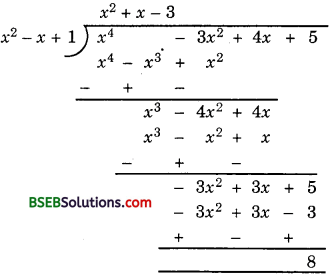∴ The quotient is x2 + x – 3 and the remainder is 8.

3. To carry out the division, we first write divisor in the standard form.
So, divisor = – x2 + 2
We have:∴ The quotient is – x2 – 2 and the remainder is – 5x + 10.Question 2.
Check whether the first polynomial is a factor of the second polynomial by dividing the second polynomial by the first polynomial:

1. t2 – 3, 2t4 + 3t3 – 2t2 – 9t – 12
2. x2 + 3x + 1, 3x4 + 5x3 – 7x2 + 2x + 2
3. x3 – 3x + 1, x5 – 4x3 + x2 + 3x + 1

Solution:
1. Let us divide 2t4 + 3t3 – 2t2 – 9t – 12 by t2 – 3.
We have: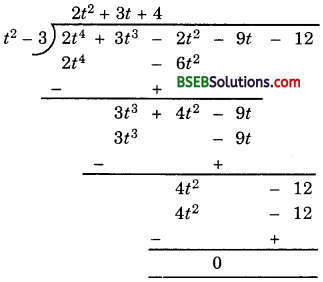Since the remainder is 0, therefore t2 – 3 is a factor of 2t4 + 3t3 – 2t2 – 9t – 12.

2. Let us divide 3x4 + 5x3 – 7x2 + 2x + 2 by x2 + 3x + 1.
We get,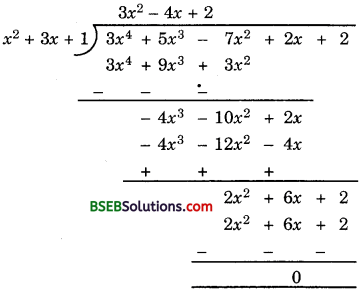Since, the remainder is 0, therefore x2 + 3x + 1 is a factor of 3x4 + 5x3 – 7x2 + 2x + 2

3. Let us divide x5 – 4x3 + x2 + 3x + 1 by x3 – 3x + 1. We get,Here, remainder is 2(≠ 0). Therefore, x3 – 3x + 1 is not a factor of
x5 – 4x3 + x2 + 3x + 1.Question 3.
Obtain all the zeroes of 3x4 + 6x3 – 2x2 – 10x – 5, if two of its zeroes are
$$\sqrt{\frac{5}{3}}$$ and – $$\sqrt{\frac{5}{3}}$$.
Solution:
Since two zeroes are $$\sqrt{\frac{5}{3}}$$ and – $$\sqrt{\frac{5}{3}}$$, so (x – $$\sqrt{\frac{5}{3}}$$) and (x + $$\sqrt{\frac{5}{3}}$$) are the factors of the given polynomial.
Now, (x – $$\sqrt{\frac{5}{3}}$$) (x + $$\sqrt{\frac{5}{3}}$$) = x2 – $$\frac{5}{3}$$.
So, (3x2 – 5) is a factor of the given polynomial.
Applying the division algorithm to the given polynomial and 3x2 – 5, we have: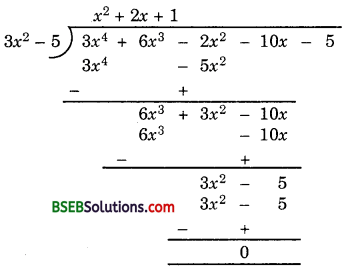∴ 3x4 + 6x3 – 2x2 – 10x – 5 = (3x2 – 5)(x2 + 2x + 1)
Now, x2 + 2x + 1 = x2 + x + x + 1
= x(x + 1) + 1(x + 1)
= (x + 1)(x + 1)
So, its other zeroes are – 1 and – 1.
Thus, all the zeroes of the given fourth degree polynomial are $$\sqrt{\frac{5}{3}}$$, – $$\sqrt{\frac{5}{3}}$$, – 1 and – 1.Question 4.
On dividing x3 – 3x2 + x + 2 by a polynomial g(x), the quoitent and remainder were x – 2 and – 2x + 4 respectively. Find g(x).
Solution:
Since on dividing x3 – 3x2 + x + 2 by a polynomial g(x), the quoitent and remainder were (x – 2) and (- 2x + 4) respectively, therefore
Quoitent × Divisor + Remainder = Dividend
or (x – 2) × g(x) + (- 2x + 4) = x3 – 3x2 + x + 2
or (x – 2) × g(x) = x3 – 3x2 + x + 2 + 2x – 4
or g(x) = $$\frac{x^{3}-3 x^{2}+3 x-2}{x-2}$$ …………… (1)
Let us divide x3 – 3x2 + 3x – 2 by x – 2. We get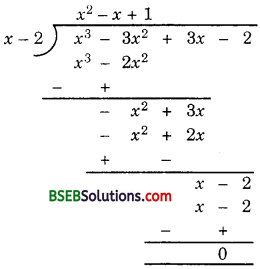∴ (1) gives g(x) = x2 – x + 1.Question 5.
Give examples of polynomials p(x), g(x), q(x) and r(x), which satisfy the division algorithm and

1. deg p(x) = deg q(x)
2. deg q(x) = deg r(x)
3. deg r(x) = 0

Solution:
There can be several examples for each of (i), (ii) and (iii).
However, one example for each case may be taken as under:

1. p(x) = 14, g(x)= 2, q(x) = x2 – x + 7, r(x) = 0
2. p(x) = x3 + x2 + x + 1, g(x) = x2 – 1, q(x) = x + 1, r(x) = 2x + 2
3. p(x) = x3 + 2x2 – x + 2, g(x) = – 1, q(x) = x + 2, r(x) = 4